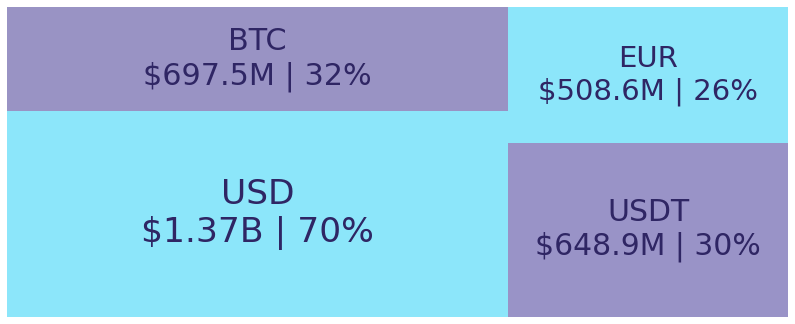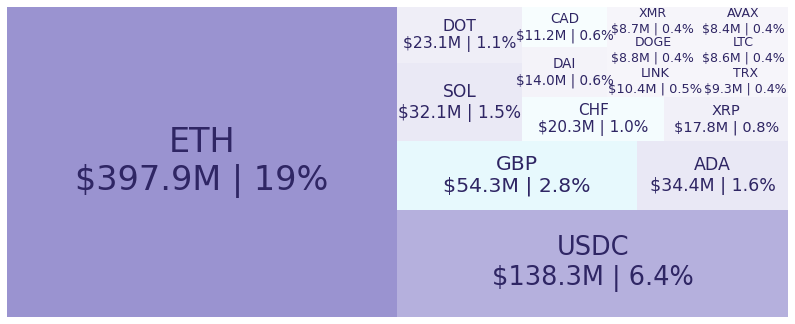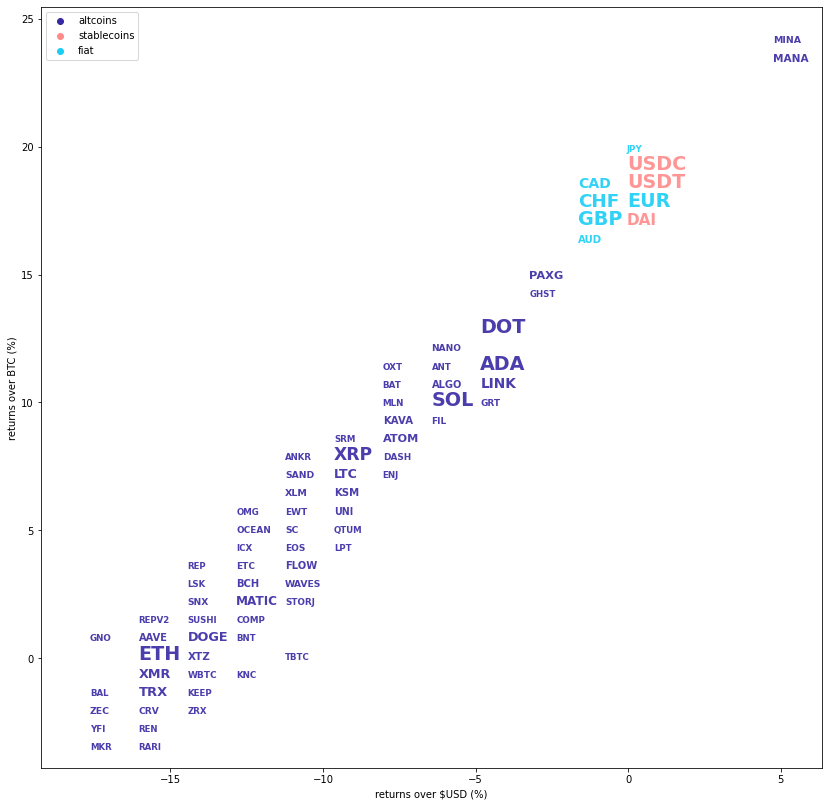# Kraken Daily Market Report for June 13 2022## Overview

• Daily spot trading volume: $2.06 billion, 30 day average spot:$668.7 million.
• Total futures notional: $631.8 million. • The top five traded coins were, respectively, Bitcoin (↓15%), Tether (↓0.02%), Ethereum (↓15%), USDC (↓0.01%), Cardana (↓5.2%). • Many coins had losses, but there were several exceptions, including Kintsugi (↑13%), WOO (↑11%), and Tribe (↑9.4%). June 13, 2022$2.06B traded across all markets today

Crypto, EUR, USD, JPY, CAD, GBP, CHF, AUD
BTC

$22460.10 ↓15%$697.5M
USDT

$1.00 ↓0.02%$648.9M
ETH

$1210.21 ↓15%$397.9M
USDC

$1.00 ↓0.01%$138.3M

$0.46 ↓5.2%$34.4M
SOL

$28.56 ↓6.5%$32.1M
DOT

$7.03 ↓4.8%$23.1M
XRP

$0.31 ↓9.3%$17.8M
DAI

$1.00 ↑0.02%$14.0M

$5.92 ↓5.6%$10.4M
TRX

$0.06 ↓16%$9.28M
DOGE

$0.05 ↓15%$8.81M
XMR

$132.56 ↓17%$8.66M
LTC

$43.41 ↓9.3%$8.56M
AVAX

$16.13 ↓6.7%$8.41M
MATIC

$0.43 ↓13%$7.16M
PAXG

$1823.36 ↓3.0%$5.81M
ATOM

$6.51 ↓7.9%$5.64M
MANA

$0.83 ↑5.2%$4.11M
XTZ

$1.40 ↓14%$3.9M
KAVA

$1.75 ↓7.8%$3.39M
KSM

$46.08 ↓9.3%$3.22M
FLOW

$1.59 ↓11%$3.02M
BCH

$126.77 ↓13%$2.9M
AAVE

$59.85 ↓15%$2.87M
ALGO

$0.30 ↓6.6%$2.8M
SHIB

$0.00001 ↓7.2%$2.64M
APE

$3.48 ↓11%$2.52M
UNI

$3.66 ↓9.8%$2.0M
XLM

$0.11 ↓10%$1.93M
MINA

$0.66 ↑5.1%$1.82M
LUNA

$0.00006 ↓13%$1.78M
ZEC

$65.49 ↓17%$1.75M
LUNA2

$2.54 ↓2.4%$1.6M
WAVES

$4.81 ↓12%$1.6M
SAND

$0.85 ↓9.8%$1.37M
PERP

$0.65 ↓11%$1.26M
SNX

$1.91 ↓14%$1.22M
SC

$0.004 ↓12%$1.13M
CRV

$0.72 ↓17%$1.1M
GLMR

$0.86 ↓13%$1.06M
MOVR

$14.65 ↓5.1%$1.05M
WBTC

$22471.70 ↓15%$1.02M
EWT

$2.41 ↓11%$907K
EOS

$0.92 ↓11%$906K
NANO

$0.85 ↓7.1%$885K
GRT

$0.11 ↓4.5%$694K
DASH

$42.47 ↓8.0%$628K
OCEAN

$0.19 ↓11%$625K
FIL

$5.31 ↓6.4%$621K
ICP

$5.42 ↓5.3%$604K
ETC

$14.39 ↓13%$570K
MLN

$21.64 ↓7.7%$544K
ICX

$0.26 ↓12%$532K
COMP

$35.64 ↓13%$526K
BAT

$0.28 ↓7.4%$517K
GALA

$0.06 ↑3.8%$505K
YFI

$4933.00 ↓17%$499K
ENJ

$0.45 ↓9.0%$477K
STORJ

$0.37 ↓13%$398K
DYDX

$1.15 ↓8.6%$392K
AXS

$13.41 ↓7.0%$388K
GNO

$125.87 ↓17%$359K
KNC

$1.23 ↓13%$356K
LRC

$0.38 ↓6.7%$353K
KEEP

$0.17 ↓16%$332K
OXT

$0.09 ↓7.4%$321K
MKR

$750.60 ↓18%$293K
FTM

$0.24 ↑6.2%$279K
SGB

$0.024 ↓13%$271K
OMG

$1.88 ↓12%$267K
LSK

$0.94 ↓14%$252K
RUNE

$1.81 ↓19%$233K
QNT

$49.74 ↑0.26%$231K
IMX

$0.70 ↓4.8%$229K
QTUM

$2.78 ↓10%$210K
ANT

$1.32 ↓6.3%$201K
CHZ

$0.09 ↓6.2%$200K
CTSI

$0.16 ↓19%$196K
REP

$6.76 ↓14%$187K
UST

$0.008 ↓16%$159K
ZRX

$0.25 ↓15%$143K
SCRT

$0.96 ↓6.2%$140K
KILT

$0.53 ↓5.0%$134K
REN

$0.09 ↓17%$124K
SUSHI

$0.97 ↓14%$120K
ASTR

$0.048 ↓1.9%$108K
LPT

$8.16 ↓10%$108K
SRM

$0.87 ↓9.1%$103K
RARI

$1.96 ↓16%$99.7K
KP3R

$95.40 ↓7.3%$93.5K
LDO

$0.62 ↓21%$87.1K
RNDR

$0.40 ↓23%$86.9K
MIR

$0.18 ↓12%$80.4K
ACA

$0.28 ↑4.5%$76.6K
GHST

$1.36 ↓4.0%$75.2K
GMT

$0.59 ↓12%$69.7K
OGN

$0.19 ↓7.8%$69.0K
1INCH

$0.60 ↓8.6%$68.4K
CQT

$0.11 ↑3.9%$67.8K
INJ

$1.32 ↓15%$67.0K
ANKR

$0.026 ↓10%$63.2K
BAL

$4.90 ↓18%$63.0K
BAND

$1.32 ↓6.1%$57.9K
ENS

$7.82 ↓7.5%$57.8K
JASMY

$0.010 ↑3.1%$51.4K
KIN

$0.00001 ↓4.7%$41.3K
GARI

$0.61 ↑2.3%$41.1K
ALICE

$2.03 ↓6.7%$40.5K
PLA

$0.32 ↓18%$37.6K
REPV2

$6.48 ↓15%$37.5K
AUDIO

$0.30 ↓8.1%$33.6K
STEP

$0.08 ↑7.2%$33.2K
SAMO

$0.004 ↓7.0%$33.2K
SDN

$0.29 ↓4.6%$32.6K
BNC

$0.22 ↓7.5%$31.8K
BNT

$0.86 ↓14%$31.4K
ATLAS

$0.006 ↑1.7%$30.8K
GST

$0.24 ↓21%$30.5K
BIT

$0.43 ↓6.5%$29.0K
PHA

$0.10 ↓5.3%$27.2K
SPELL

$0.0008 ↓2.9%$27.1K
PSTAKE

$0.12 ↓36%$24.0K

$3.35 ↓19%$22.9K
AKT

$0.28 ↓7.8%$22.8K
MC

$0.78 ↓12%$21.8K
KINT

$3.83 ↑13%$18.7K
KAR

$0.43 ↑0.7%$17.7K
FXS

$4.77 ↓11%$17.5K
CFG

$0.24 ↓1.3%$16.5K
FET

$0.11 ↓6.9%$16.4K
COTI

$0.09 ↓11%$15.4K
NYM

$0.30 ↑1.6%$14.2K
TLM

$0.030 ↓33%$13.2K
XRT

$4.00 ↓4.0%$12.2K
CHR

$0.18 ↓35%$12.1K
BOND

$2.79 ↓17%$12.1K
ORCA

$0.69 ↓5.1%$12.0K
FIDA

$0.33 ↓9.3%$11.6K
ACH

$0.013 ↓0.32%$10.7K
RBC

$0.06 ↓12%$10.2K
OXY

$0.08 ↓1.0%$8.3K
WOO

$0.13 ↑11%$7.54K
TBTC

$24001.00 ↓11%$7.05K
REQ

$0.09 ↑8.8%$6.5K
IDEX

$0.06 ↓10%$5.86K
RAY

$0.69 ↓2.1%$5.8K

$0.036 ↓16%$5.56K
CVX

$5.36 ↓6.8%$5.13K
MV

$0.46 ↓4.7%$5.13K
API3

$1.17 ↓0.3%$4.72K
SUPER

$0.14 ↓9.9%$4.43K
AIR

$0.019 ↓15%$4.32K
YGG

$0.39 ↓12%$4.23K
RLC

$0.70 ↓3.4%$4.11K

$0.17 ↓58%$3.85K
ALCX

$21.19 ↓12%$3.79K
MULTI

$3.41 ↓9.8%$2.75K
RARE

$0.17 ↓1.4%$2.63K
TVK

$0.033 ↓2.1%$2.61K
AGLD

$0.30 ↓14%$2.61K

$1.28 ↓5.2%$2.56K
ROOK

$28.32 ↓28%$2.49K
BICO

$0.48 ↑1.7%$2.48K
SBR

$0.006 ↓6.6%$2.41K
MNGO

$0.046 ↓1.7%$2.18K
UMA

$2.03 ↓7.6%$2.15K
POLIS

$0.42 ↓4.6%$1.75K
POWR

$0.19 ↓7.7%$1.69K
TRIBE

$0.20 ↑9.4%$1.67K
CVC

$0.14 ↓2.0%$1.54K

$1.60 ↓3.6%$1.37K
NMR

$8.59 ↓12%$1.17K
TOKE

$1.98 ↓14%$0.92K
MSOL

$30.11 ↓2.9%$0.32K

#####################. Trading Volume by Asset. ##########################################

## Trading Volume by Asset

The figures below break down the trading volume of the largest, mid-size, and smallest assets. Cryptos are in purple, fiats are in blue. For each asset, the chart contains the daily trading volume in USD, and the percentage of the total trading volume. The percentages for fiats and cryptos are treated separately, so that they both add up to 100%.

Figure 1: Largest trading assets: trading volume (measured in USD) and its percentage of the total trading volume (June 13 2022)Figure 2: Mid-size trading assets: (measured in USD) (June 13 2022)###########. Daily Returns. #################################################

## Daily Returns %

Figure 3: Returns over USD and XBT. Relative volume and return size is indicated by the size of the font. (June 13 2022)###########. Disclaimer #################################################

The values generated in this report are from public market data distributed from Kraken WebSockets api. The total volumes and returns are calculated over the reporting day using UTC time.